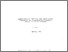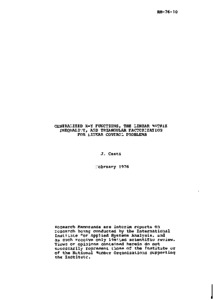Generalized X-Y Functions, the Linear Matrix Inequality, and Triangular Factorization for Linear Control Problems

Casti, J.L. (1976). Generalized X-Y Functions, the Linear Matrix Inequality, and Triangular Factorization for Linear Control Problems. IIASA Research Memorandum. IIASA, Laxenburg, Austria: RM-76-010Preview
Text
RM-76-010.pdf

Abstract

The relationship between the linear matrix inequality (LMI), generalized X-Y functions, and triangular factorization is examined within the framework of the classical linear-quadratic-gaussian problem. It is shown that the generalized X-Y functions arise naturally as components within the factors of the matrix forming the LMI when that matrix is decomposed into its symmetric triangular factors. This viewpoint enables us to propose a low-dimensional computational algorithm for time-dependent problems which reduces to the generalized X-Y situation for constant systems.

In addition to the basic factorization results, we also briefly touch upon several related topics including the infinite-interval (regulator) problem, singular control problems, canonical forms, and numerical considerations.

Item Type: Monograph (IIASA Research Memorandum) System and Decision Sciences - Core (SDS) IIASA Import 15 Jan 2016 01:43 27 Aug 2021 17:08 http://pure.iiasa.ac.at/674View Item